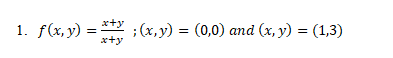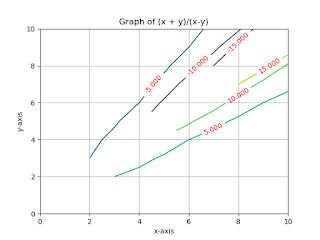Limits and Continuity of Two or More Variables

## Limits and Continuity in Mathematics

To develop a calculus for functions of a variable, we needed to build an understanding of the concept of a limit, which we needed to understand continuous functions and define derivations. The extent to which the functions of two variables can be included can be difficult to a large extent; Fortunately, most of the work we do is fairly easy to understand.

The potential difficulty is largely due to the fact that there are many ways to "approach" a point in the XY plane. If we want to say that

we need to hold the idea that like (x, y) close to (a, b), Then f (x, y) becomes closer to L. For functions of a variable, f (x), there are only two ways that x can approach: from the left or right. But (a, b) has infinite ways to approach it: along with any one of the infinite lines, or an infinite number of parables or infinity. The number of sine curves, and so on.

We might expect that this is not really so bad - for example, that along every possible line the value of (a, b) becomes closer to L than the value of f (x, y); This means that "f (x, y) approaches L as (x, y) approaches (A, B) '". Sadly no.

Now Let's take look at how to check Limits and Continuity of Functions of Two or More Variables in Python.

1. Draw the following functions and discuss whether limit exits or not as (x,y) approaches to the given points.`Python Code:`
```import matplotlib.pyplot as plt
import numpy as np
x = np.linspace(0,10,11)
y = np.linspace(0,10,11)
x, y = np.meshgrid(x, y)
z = (x+y)/(x-y)
for i in range(z.shape):
for j in range(z.shape):
if i==0 and j==0:
print("limit doesnot exits at (0,0)")
pass        elif(z[i,j]==np.inf or z[i,j]==np.float64(np.nan)):
if i ==1 and j ==3:
print("limit doesnot exists at (1,3)")
else:
cs = plt.contour(x,y,z)
plt.clabel(cs, inline = 1,fontsize= 10,colors ='r')
plt.grid(True)
plt.xlabel('x-axis')
plt.ylabel('y-axis')
plt.title('Graph of (x + y)/(x-y)')
plt.show()
```Limits and Continuity of Functions of Two or More Variables in python ``` 2. Draw the following functions and discuss whether limit exits or not as (x,y) approaches to the given points.Python Code: import matplotlib.pyplot as plt import numpy as np x = np.linspace(0,10,11) y = np.linspace(0,10,11) x, y = np.meshgrid(x, y) z = (x-y)/(np.sqrt(x**2+y**2)) for i in range(z.shape): for j in range(z.shape): if i==0 and j==0: print("limit doesnot exits at (0,0)") pass elif(z[i,j]==np.inf or z[i,j]==np.float64(np.nan)): if i ==2 and j ==1: print("limit doesnot exists at (2,1)") else: cs = plt.contour(x,y,z) plt.clabel(cs, inline = 1,fontsize= 10,colors ='r') plt.grid(True) plt.xlabel('x-axis') plt.ylabel('y-axis') plt.title('Graph of (x -y)/np.sqrt(x**2+y**2)') plt.show()Limits and Continuity of Functions of Two or More Variables in python ```